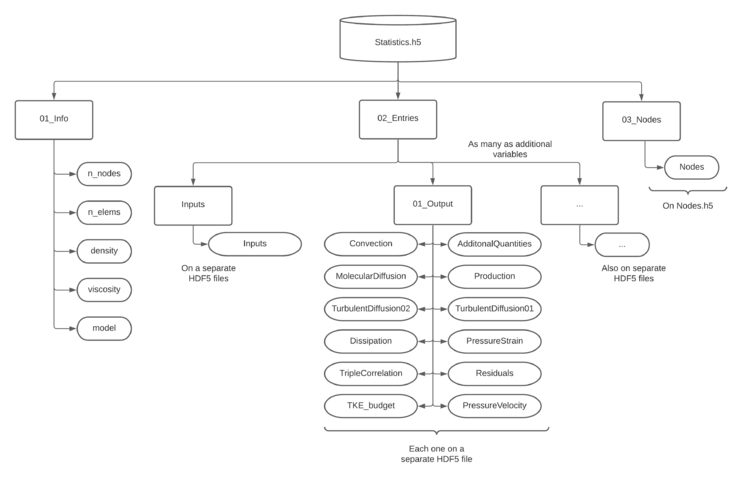# Storage Format

The data provided is stored in HDF5 format. This can be easily read through the HDF5 library or python's h5py. Any parallel partition has been taken out from the dataset for easier reading both in serial and parallel.

### Notes on the HDF5 library

The following instructions are intended for users who wish to compile and obtain the parallel h5py package. Note that the serial h5py also works, however, its parallel capabilities will be deactivated.

manual install

The package h5py can be manually installed with parallel support provided the right libraries are in the system. To get them use (on a Debian-like distribution):

sudo apt install libhdf5-mpi-dev


or make sure that the environment variable HDF5_DIR is pointing to your hdf5 installation. Then install h5py from pip using:

CC=mpicc HDF5_MPI="ON" pip install --no-binary=h5py h5py


## Instantaneous data format

The dataset consists of a single file per snapshot, directly containing all the variables as well as the node positions. It also contains some metadata elements such as the number of points, current simulation time and instant. The names of the provided variables are:

• xyz, are the node positions as an array of (npoints,3).
• PRESS, is the instantaneous pressure as a scalar array of (npoints,).
• VELOC, is the instantaneous velocity as a vectorial array of (npoints,3).
• GRADP, is the gradient of pressure as a vectorial array of (npoints,3).
• GRADV, is the gradient of velocity as a tensorial array of (npoints,9).

### Reading the data with python

An example on how to read this dataset in python follows:

import h5py, numpy as np

filename = 'duct_0.h5' # duct area, first snapshot

# Open HDF5 file in serial
file     = h5py.File(filename,'r')

PRESS = np.array(file['PRESS'],dype=np.double)
VELOC = np.array(file['VELOC'],dype=np.double)

# Close file
file.close()


## Statistical data format

The dataset consists of a master file which links a number of external files, thus creating a tree-like database. Each of these external files contain an array of a certain number of positions (1 if scalar, 3 if vectorial and 6 if tensorial, with the exception of the velocity triple correlation). A graphical representation of the database is provided in Fig. 26.Figure 26: Schematic representation of the HDF5 statistical database.

The data is structured in different external files, as shown in Fig. 26. The list number minus 1 corresponds to the array position on python (since python starts counting on 0).

Inputs

1. ${\overline {P}}$2. ${\overline {U}}$3. ${\overline {V}}$4. ${\overline {W}}$5. ${{\overline {\tau }}_{11}}$6. ${{\overline {\tau }}_{12}}$7. ${{\overline {\tau }}_{22}}$8. ${{\overline {\tau }}_{13}}$9. ${{\overline {\tau }}_{23}}$10. ${{\overline {\tau }}_{33}}$11. ${R_{11}}$12. ${R_{12}}$13. ${R_{22}}$14. ${R_{13}}$15. ${R_{23}}$16. ${R_{33}}$17. ${\partial {\overline {P}}/\partial x}$18. ${\partial {\overline {U}}/\partial x}$19. ${\partial {\overline {V}}/\partial x}$20. ${\partial {\overline {W}}/\partial x}$21. ${\partial {\overline {P}}/\partial y}$22. ${\partial {\overline {U}}/\partial y}$23. ${\partial {\overline {V}}/\partial y}$24. ${\partial {\overline {W}}/\partial y}$25. ${\partial {\overline {P}}/\partial z}$26. ${\partial {\overline {U}}/\partial z}$27. ${\partial {\overline {V}}/\partial z}$28. ${\partial {\overline {W}}/\partial z}$1. ${\overline {pp}}$2. ${\eta _{T}}$3. ${\eta _{K}}$4. ${\eta _{tK}}$Triple Correlation

1. ${\rho {\overline {uuu}}}$2. ${\rho {\overline {uvu}}}$3. ${\rho {\overline {vvu}}}$4. ${\rho {\overline {uwu}}}$5. ${\rho {\overline {vwu}}}$6. ${\rho {\overline {wwu}}}$7. ${\rho {\overline {vvv}}}$8. ${\rho {\overline {vwv}}}$9. ${\rho {\overline {wwv}}}$10. ${\rho {\overline {www}}}$Pressure Velocity Correlation

1. ${\overline {pu}}$2. ${\overline {pv}}$3. ${\overline {pw}}$Budget Equation Components The components of the Reynolds stress budget equation come in the following order (for a generic budget component $\phi$):

1. ${\phi _{11}}$2. ${\phi _{12}}$3. ${\phi _{22}}$4. ${\phi _{13}}$5. ${\phi _{23}}$6. ${\phi _{33}}$### Reading the data with python

The following script is provided to facilitate the reading of the dataset. An example on how to use this script follows:

from HiFiTurbDB_Reader import HiFiTurbDB_Reader

db  = HiFiTurbDB_Reader(FILENAME,return_matrix=True,parallel=False) # Return outputs as matrices
print(db,flush=True) # We can print the database information

# Recover some variables
xyz           = db.points
Rij           = db.reynolds_stress   # Reynolds stresses


Alternatively, the dataset can be read raw by directly using the h5py library. An example follows:

import h5py, numpy as np

file = h5py.File('Statistics.h5','r')

xyz  = np.array(file['03_Nodes']['Nodes'],dype=np.double)